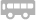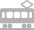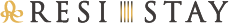## cotorune KYOTO

-

1楼大厅有可以自由使用的共享厨房。

##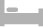房间介绍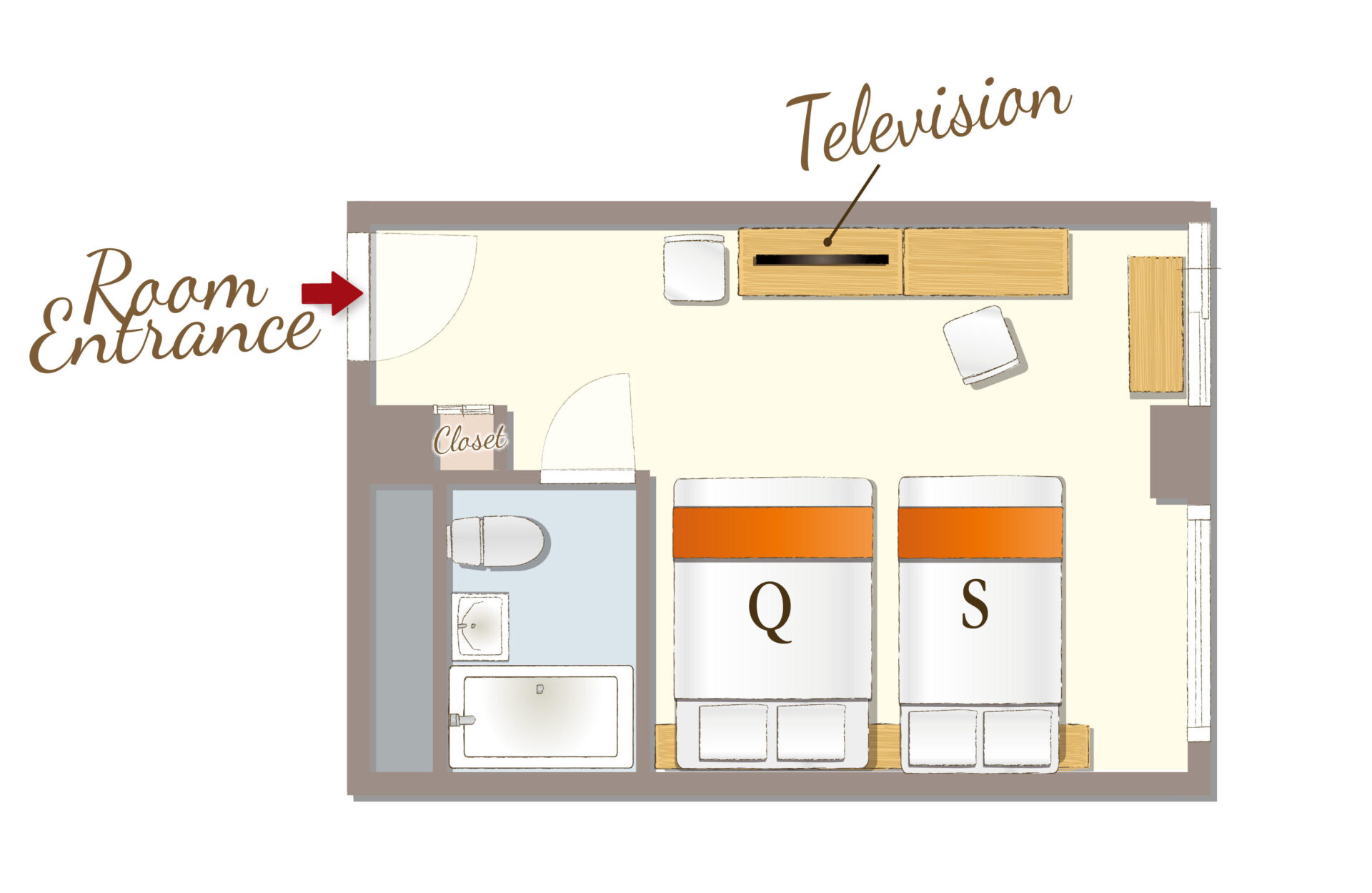## Standard Triple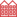公寓式

[可容纳人数 : 3 位]
・单人床(幅 1,000mm) ×1张
・大床(幅 1,600mm) × 1张

• 温水便座
• 浴缸
• 吹风机
• 空调
• 保险柜
• TV
• AppleTV/androidTV
• Netflix
• USB充电插口
• Wi-Fi
• 冰箱
• 电水壶
• 衣架
• 电熨斗
• 壁橱
• 吸尘器
• 禁烟

• 洗发水
• 护发素
• 沐浴液
• 洗脸毛巾
• 浴巾
• 浴室防滑垫
• 拖鞋
• 化妆棉
• 牙刷
• 化妆棉
• 沐浴剂
• 便笺/笔
• 咖啡/茶/绿茶
• 矿泉水
• 梳子## Superior Triple公寓式

[可容纳人数 : 3 位]
・单人床(幅 1,000mm) ×1张
・大床(幅 1,600mm) × 1张

• 温水便座
• 浴缸
• 吹风机
• 空调
• 保险柜
• TV
• AppleTV/androidTV
• Netflix
• USB充电插口
• Wi-Fi
• 冰箱
• 电水壶
• 衣架
• 电熨斗
• 壁橱
• 吸尘器
• 禁烟

• 洗发水
• 护发素
• 沐浴液
• 洗脸毛巾
• 浴巾
• 浴室防滑垫
• 拖鞋
• 化妆棉
• 牙刷
• 化妆棉
• 沐浴剂
• 便笺/笔
• 咖啡/茶/绿茶
• 矿泉水
• 梳子## Standard Triple with Kitchen公寓式

[可容纳人数 : 3 位]
・单人床(幅 1,000mm) ×1张
・大床(幅 1,600mm) × 1张

• 温水便座
• 浴缸
• 吹风机
• 空调
• 保险柜
• TV
• AppleTV/androidTV
• Netflix
• USB充电插口
• Wi-Fi
• 冰箱
• 微波炉
• 电水壶
• 電磁爐
• 烹调器具
• 餐具
• 衣架
• 电熨斗
• 壁橱
• 吸尘器
• 禁烟

• 洗发水
• 护发素
• 沐浴液
• 洗脸毛巾
• 浴巾
• 浴室防滑垫
• 拖鞋
• 化妆棉
• 牙刷
• 化妆棉
• 沐浴剂
• 便笺/笔
• 咖啡/茶/绿茶
• 矿泉水
• 梳子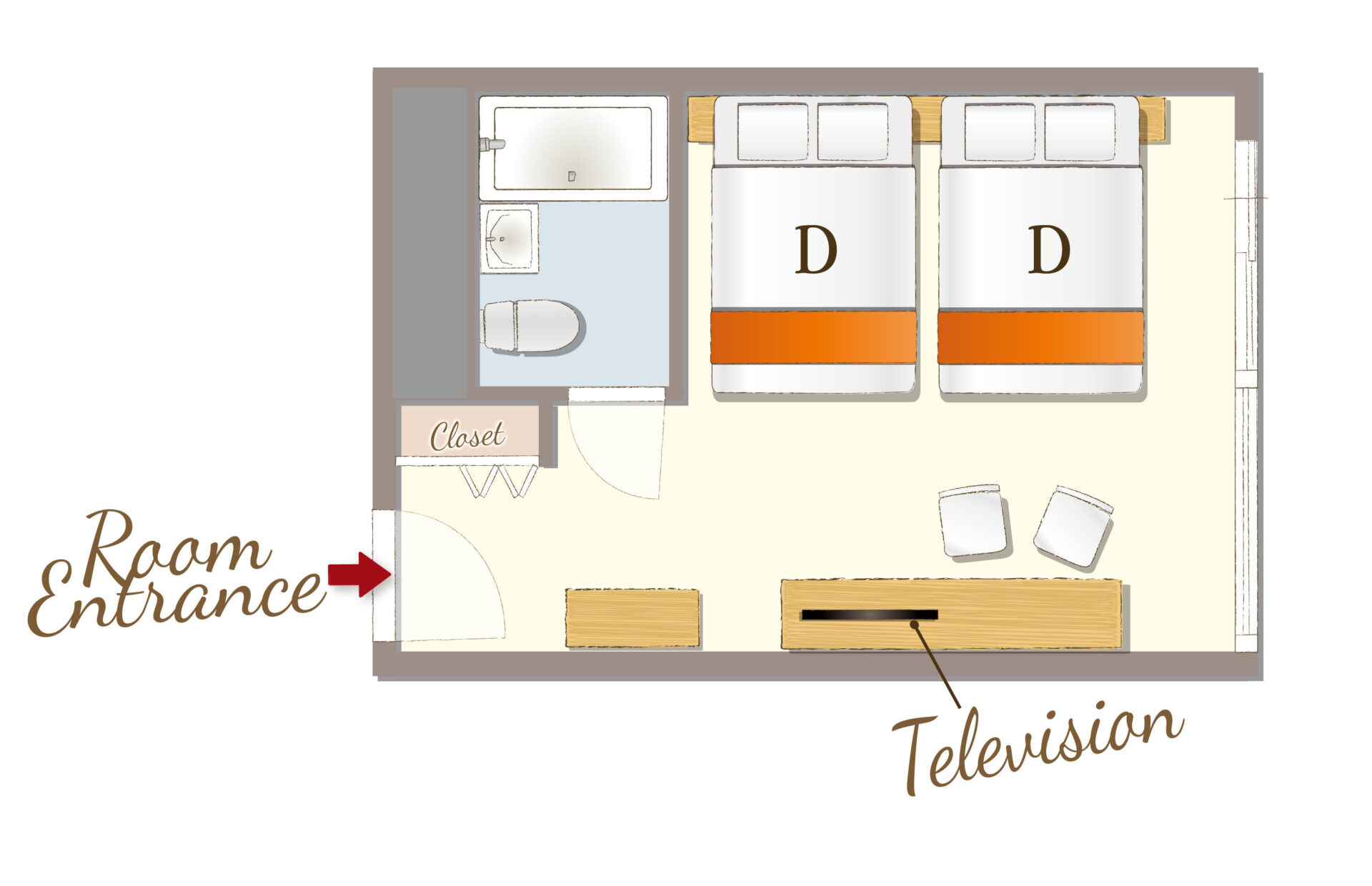## Standard Quad公寓式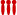可供4位以上入住

[可容纳人数 : 4 位]
・双人床(幅 1,400mm) × 2张

• 温水便座
• 浴缸
• 吹风机
• 空调
• 保险柜
• TV
• AppleTV/androidTV
• Netflix
• USB充电插口
• Wi-Fi
• 冰箱
• 电水壶
• 衣架
• 电熨斗
• 壁橱
• 吸尘器
• 禁烟

• 洗发水
• 护发素
• 沐浴液
• 洗脸毛巾
• 浴巾
• 浴室防滑垫
• 拖鞋
• 化妆棉
• 牙刷
• 化妆棉
• 沐浴剂
• 便笺/笔
• 咖啡/茶/绿茶
• 矿泉水
• 梳子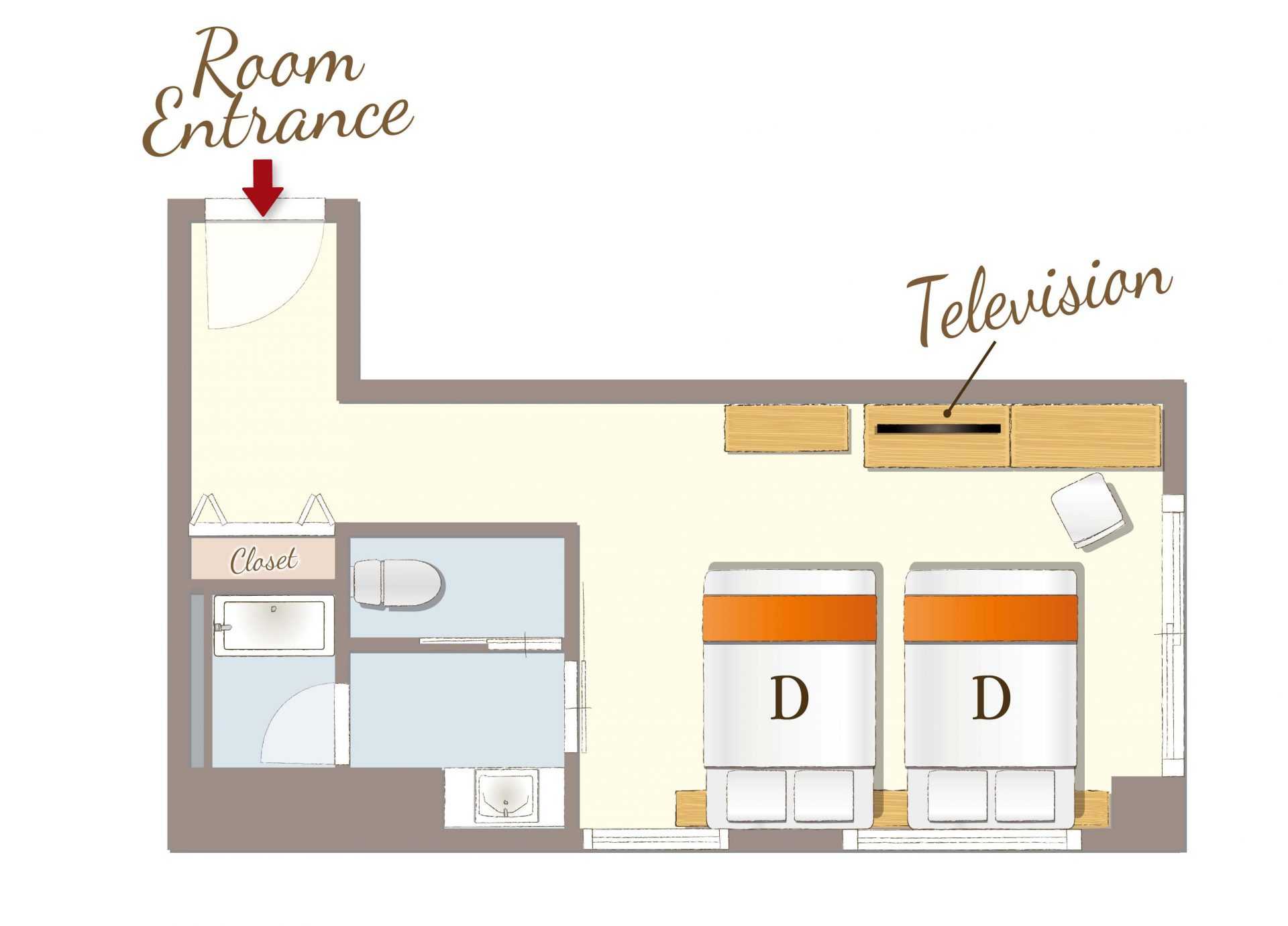## Superior Quad公寓式可供4位以上入住

[可容纳人数 : 4 位]
・双人床(幅 1,400mm) × 2张

• 温水便座
• 浴缸
• 吹风机
• 空调
• 保险柜
• TV
• AppleTV/androidTV
• Netflix
• USB充电插口
• Wi-Fi
• 冰箱
• 电水壶
• 衣架
• 电熨斗
• 壁橱
• 吸尘器
• 禁烟

• 洗发水
• 护发素
• 沐浴液
• 洗脸毛巾
• 浴巾
• 浴室防滑垫
• 拖鞋
• 化妆棉
• 牙刷
• 化妆棉
• 沐浴剂
• 便笺/笔
• 咖啡/茶/绿茶
• 矿泉水
• 梳子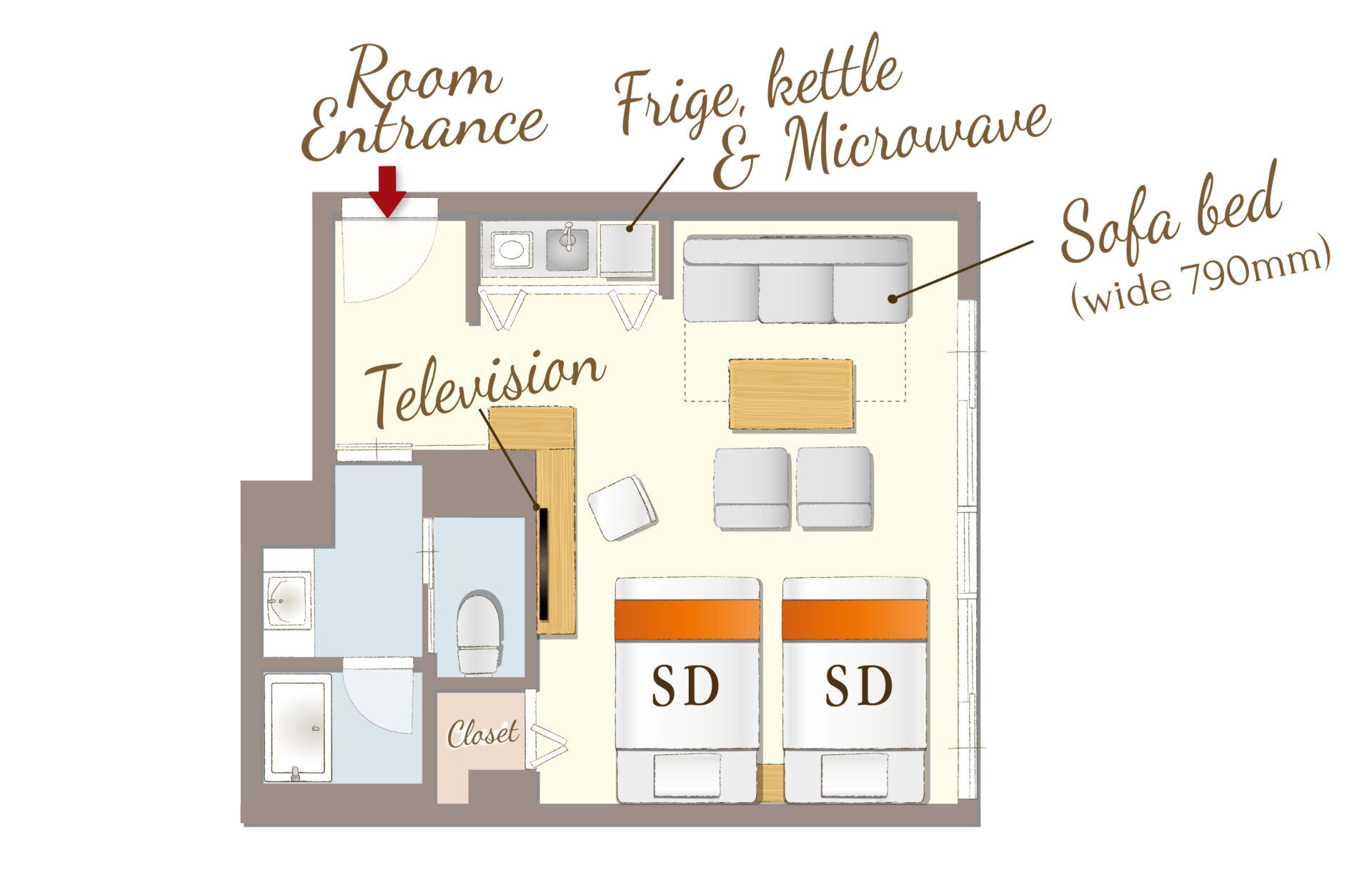## Superior Triple with Kitchen公寓式

[可容纳人数 : 3 位]
・半双人床(幅 1,200mm) × 2张
・沙发床 × 1张

• 温水便座
• 浴缸
• 吹风机
• 空调
• 保险柜
• TV
• AppleTV/androidTV
• Netflix
• USB充电插口
• Wi-Fi
• 冰箱
• 微波炉
• 电水壶
• 電磁爐
• 烹调器具
• 餐具
• 衣架
• 电熨斗
• 壁橱
• 吸尘器
• 禁烟

• 洗发水
• 护发素
• 沐浴液
• 洗脸毛巾
• 浴巾
• 浴室防滑垫
• 拖鞋
• 化妆棉
• 牙刷
• 化妆棉
• 沐浴剂
• 便笺/笔
• 咖啡/茶/绿茶
• 矿泉水
• 梳子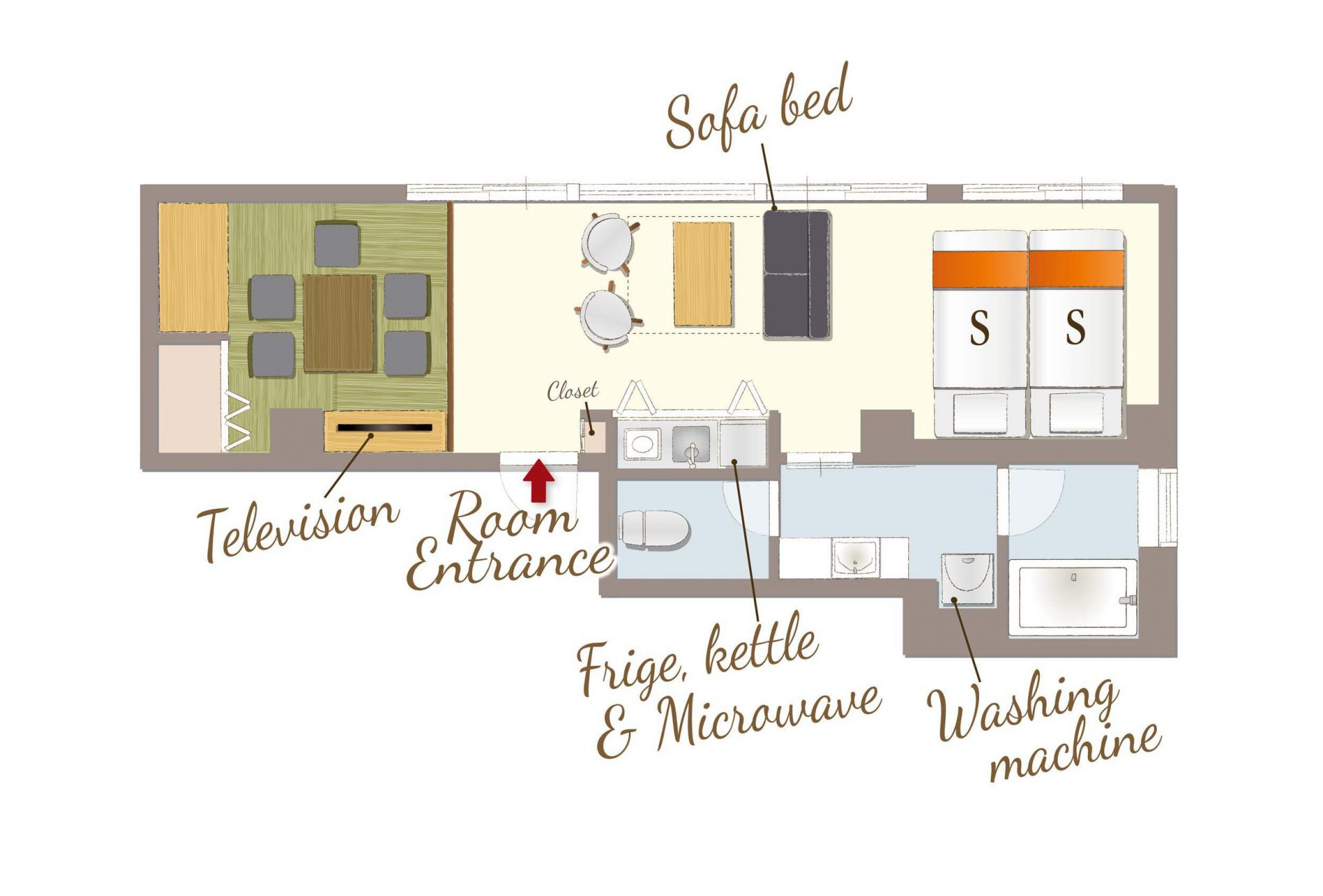## Japanese Quad with Kitchen公寓式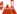配有日式客房的住宿设施可供4位以上入住

[可容纳人数 : 4 位]
・单人床(幅 1,000mm) ×2张
・沙发床× 1张
・日式蒲团(幅 970mm) × 1张

• 温水便座
• 浴缸
• 吹风机
• 空调
• 保险柜
• TV
• AppleTV/androidTV
• Netflix
• USB充电插口
• Wi-Fi
• 冰箱
• 微波炉
• 电水壶
• 電磁爐
• 烹调器具
• 餐具
• 洗衣机
• 衣架
• 电熨斗
• 壁橱
• 吸尘器
• 禁烟

• 洗发水
• 护发素
• 沐浴液
• 洗脸毛巾
• 浴巾
• 浴室防滑垫
• 拖鞋
• 化妆棉
• 牙刷
• 化妆棉
• 沐浴剂
• 便笺/笔
• 咖啡/茶/绿茶
• 矿泉水
• 梳子## Superior Family with Kitchen公寓式可供4位以上入住

[可容纳人数 : 5 位]
・双人床(幅 1,400mm) × 2张
・沙发床× 1张

• 温水便座
• 浴缸
• 吹风机
• 空调
• 保险柜
• TV
• AppleTV/androidTV
• Netflix
• USB充电插口
• Wi-Fi
• 冰箱
• 微波炉
• 电水壶
• 電磁爐
• 烹调器具
• 餐具
• 洗衣机
• 衣架
• 电熨斗
• 壁橱
• 吸尘器
• 禁烟

• 洗发水
• 护发素
• 沐浴液
• 洗脸毛巾
• 浴巾
• 浴室防滑垫
• 拖鞋
• 化妆棉
• 牙刷
• 化妆棉
• 沐浴剂
• 便笺/笔
• 咖啡/茶/绿茶
• 矿泉水
• 梳子## Japanese Family Suite with Kitchen公寓式配有日式客房的住宿设施可供4位以上入住

[可容纳人数 : 5 位]
・半双人床(幅 1,200mm) × 2张
・日式蒲团(幅 970mm) × 3张

• 温水便座
• 浴缸
• 吹风机
• 空调
• 保险柜
• TV
• AppleTV/androidTV
• Netflix
• USB充电插口
• Wi-Fi
• 冰箱
• 微波炉
• 电水壶
• 電磁爐
• 烹调器具
• 餐具
• 洗衣机
• 衣架
• 电熨斗
• 壁橱
• 吸尘器
• 禁烟

• 洗发水
• 护发素
• 沐浴液
• 洗脸毛巾
• 浴巾
• 浴室防滑垫
• 拖鞋
• 化妆棉
• 牙刷
• 化妆棉
• 沐浴剂
• 便笺/笔
• 咖啡/茶/绿茶
• 矿泉水
• 梳子

##交通